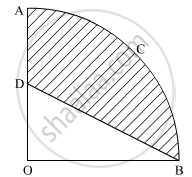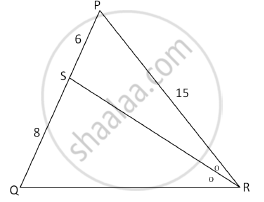Share

# Important Questions for ICSE Class 10 - CISCE - Mathematics

Course
Subjects
Topics
Subjects
Popular subjects
Topics
Mathematics
< prev 1 to 20 of 325 next >

In the given figure, OACB is a quadrant of a circle with centre O and radius 3.5 cm. If OD = 2 cm, find the area of the shaded region.Appears in 4 question papers
Chapter: [0.032] Circles
Concept: Areas of Sector and Segment of a Circle

If the quadratic equation px2 − 2√5px + 15 = 0 has two equal roots then find the value of p.

Appears in 3 question papers
Concept: Nature of Roots

In the following figure, in ΔPQR, seg RS is the bisector of ∠PRQ. If PS = 6, SQ = 8, PR = 15, find QR.Appears in 3 question papers
Chapter: [0.035] Similarity
Concept: Similarity of Triangles

Jaya borrowed Rs. 50,000 for 2 years. The rates of interest for two successive years are 12% and 15% respectively. She repays 33,000 at the end of the first year. Find the amount she must pay at the end of the second year to clear her debt.

Appears in 1 question paper
Chapter: [0.011000000000000001] Compound Interest
Concept: Conept of Compound Interest > Compound Interest as a Repeated Simple Interest Computation with a Growing Principal

Mr Lalit invested Rs. 5000 at a certain rate of interest, compounded annually for two years. At the end of the first year, it amounts to Rs. 5325. Calculate

1) The rate of interest

2) The amount at the end of the second year, to the nearest rupee.

Appears in 1 question paper
Chapter: [0.011000000000000001] Compound Interest
Concept: Use of Compound Interest in Computing Amount Over a Period of 2 Or 3-years

In what time will Rs. 1500 yield Rs. 496.50 as compound interest at 10% per annum compounded annually?

Appears in 1 question paper
Chapter: [0.011000000000000001] Compound Interest
Concept: Use of Compound Interest in Computing Amount Over a Period of 2 Or 3-years

The present population of the town is 2,00,000. The population is increased by 10% in the first year and 15% in the second year. Find the population of the town at the end of two years.

Appears in 1 question paper
Chapter: [0.011000000000000001] Compound Interest
Concept: Use of Compound Interest in Computing Amount Over a Period of 2 Or 3-years

If a, b, c are in continued proportion, prove that (a + b + c) (a – b + c) = a2 + b2 + c2

Appears in 1 question paper
Chapter: [0.011000000000000001] Compound Interest
Concept: Conept of Compound Interest > Compound Interest as a Repeated Simple Interest Computation with a Growing Principal

Ranbir borrows Rs. 20,000 at 12% per annum compound interest. If he repays Rs. 8400 at the end of the first year and Rs. 9680 at the end of the second year, find the amount of loan outstanding at the beginning of the third year.

Appears in 1 question paper
Chapter: [0.011000000000000001] Compound Interest
Concept: Use of Compound Interest in Computing Amount Over a Period of 2 Or 3-years

The compound interest, calculated yearly, on a certain sum of money for the second year is Rs. 1320 and for the third year is Rs. 1452. Calculate the rate of interest and the original sum of money

Appears in 1 question paper
Chapter: [0.011000000000000001] Compound Interest
Concept: Use of Compound Interest in Computing Amount Over a Period of 2 Or 3-years

At what rate % p.a. will a sum of Rs. 4000 yield Rs. 1324 as compound interest in 3 years?

Appears in 1 question paper
Chapter: [0.011000000000000001] Compound Interest
Concept: Conept of Compound Interest > Compound Interest as a Repeated Simple Interest Computation with a Growing Principal

On what sum of money will the difference between the compound interest and simple interest for 2 years be equal to Rs. 25 if the rate of interest charged for both is 5% p.a.?

Appears in 1 question paper
Chapter: [0.011000000000000001] Compound Interest
Concept: Conept of Compound Interest > Compound Interest as a Repeated Simple Interest Computation with a Growing Principal

Mr Kumar borrowed Rs. 15000 for two years. The rates of interest for two successive years are 8% and 10% respectively. If he repays Rs. 6200 at the end of the first year, find the outstanding amount at the end of the second year.

Appears in 1 question paper
Chapter: [0.011000000000000001] Compound Interest
Concept: Conept of Compound Interest > Compound Interest as a Repeated Simple Interest Computation with a Growing Principal

In what period of time will Rs. 12,000 yield Rs. 3972 as compound interest at 10% per annum, if compounded on a yearly basis?

Appears in 1 question paper
Chapter: [0.011000000000000001] Compound Interest
Concept: Use of Compound Interest in Computing Amount Over a Period of 2 Or 3-years

Nikita invests Rs. 6000 for two years at a certain rate of interest compounded annually. At the end of the first year, it amounts to Rs. 6720. Calculate:

1) the rate of interest.

2) the amount at the end of the second year.

Appears in 1 question paper
Chapter: [0.011000000000000001] Compound Interest
Concept: Use of Compound Interest in Computing Amount Over a Period of 2 Or 3-years

Rohit borrows Rs. 86,000 from Arun for two years at 5% per annum simple interest. He immediately lends out this money to Akshay at 5% compound interest compounded annually for the same period. Calculate Rohit’s profit in the transaction at the end of two years.

Appears in 1 question paper
Chapter: [0.011000000000000001] Compound Interest
Concept: Conept of Compound Interest > Compound Interest as a Repeated Simple Interest Computation with a Growing Principal

How much should a man invest in Rs. 50 shares selling at Rs. 60 to obtain an income of Rs. 450, if the rate of dividend declared is 10%. Also find his yield percent, to the nearest whole number.

Appears in 1 question paper
Chapter: [0.012] Shares and Dividends
Concept: Shares and Dividends Examples

Ashok invested Rs. 26,400 on 12%, Rs. 25 shares of a company. If he receives a dividend of Rs. 2,475. Find the :

1) number of shares he bought

2) The market value of each share

Appears in 1 question paper
Chapter: [0.012] Shares and Dividends
Concept: Shares and Dividends

A man invests Rs. 22,500 in Rs. 50 shares available at 10% discount. If the dividend paid by the company is 12%, calculate:

1) The number of shares purchased

3) The rate of return he gets on his investment. Give your answer correct to the nearest whole number.

Appears in 1 question paper
Chapter: [0.012] Shares and Dividends
Concept: Shares and Dividends Examples

Rohit invested Rs. 9,600 on Rs. 100 shares at Rs. 20 premium paying 8% dividend. Rohit sold the shares when the price rose to Rs 160. He invested the proceeds (excluding dividend) in 10% Rs. 50 shares at Rs. 40. Find the:

1) original number of shares

2) sale proceeds

3) new number of shares

4) change in the two dividends.

Appears in 1 question paper
Chapter: [0.012] Shares and Dividends
Concept: Shares and Dividends
< prev 1 to 20 of 325 next >
Important Questions for ICSE Class 10 CISCE Mathematics. You can further filter Important Questions by subjects and topics. Chapter wise important Questions for Class 10 CISCE. it gets easy to find all Class 10 important questions with answers in a single place for students. Saving time and can then focus on their studies and practice. Important questions for Class 10 chapter wise with solutions

Class 10 Mathematics can be very challenging and complicated. But with the right ideas and support, you will be able to make it work. That’s why we have the class 10 Mathematics important questions with answers pdf ready for you. All you need is to acquire the PDF with all the content and then start preparing for the exam. It really helps and it can bring in front an amazing experience every time if you're tackling it at the highest possible level.